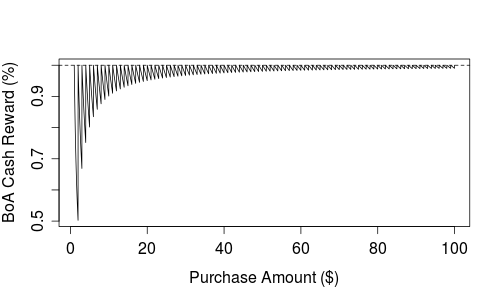Want to share your content on R-bloggers? click here if you have a blog, or here if you don't.

Thanks to Anthony Damico who alerted me to an error in the Bank of America’s 1% cash rewards figure in my last post. The plot shows the true reward percentage as a function of purchase amount. A key “feature” of the BoA reward program is that the full 1% is only awarded for purchases in whole dollar amounts. But, in the figure I neglected to plot the function at each whole dollar amount. Hence, the function was a bit misleading. Anthony sent the code below that corrects this; the figure is below.

```x <- seq(1,100,length.out=9901)
plot(x, trunc(x)/x, type="l",
xlab="Purchase Amount (\$)",
ylab="BoA Cash Reward (%)")
abline(h=1, lty=2)
```